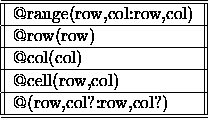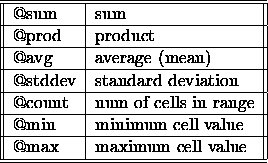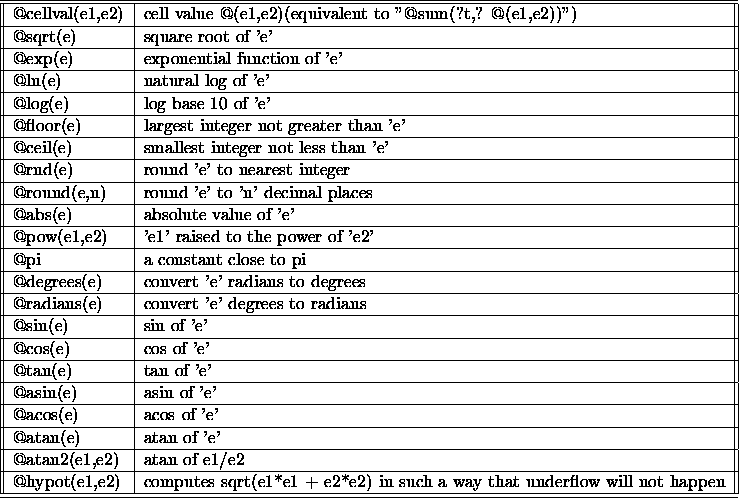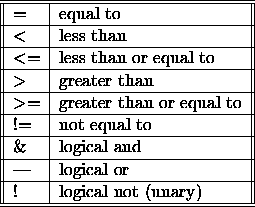Next: 8 Graphs Up: 7 Generating reports Previous: 7.8 Email Reports

# 7.9 Creating new columns

Faunix provides the ability for you to create new columns to display on a report. Please be warned, this section is for advanced users and is quite technical.

To create a new column, click the ``New'' button to the right of the ``User-defined columns'' label. The window shown in figure 7.16 is displayed.Figure 7.16: Define a new column on your report

As you can see from figure 7.16, there are a lot of built-in commands to help you construct your new column. Clicking a button on this window enters the corresponding text in the field construction window. This section describes the formulas to use in creating a user-defined column.

There are four types of subexpressions:

1. numeric constants/expressions
2. ranges
3. range functions
4. numeric functions

Ranges can only be used as arguments to a range function. Range functions take ranges as arguments and produce real numbers; numeric functions take numbers as arguments and produce real numbers. All calculations and values are represented in double precision floating point.

## 7.9.1 Numeric expressions

Numeric expressions may include any of the following operators and may be fully parenthesized. Precedence is the same as the C programming language:

```- + * / %
```

For example, the following are all valid numeric expressions:

```(2+3)/4
2+3/4
97*2+98*2/3-42.0```

## 7.9.2 Ranges

A range can be any of the following as shown in table 7.1Table 7.1: Ranges

Row and column names may be used as arguments to ranges, as well as numeric expressions and the following constants:

• @thisrow
• @thiscol
• @maxrow
• @maxcol

The constants are evaluated in the current table context (that is, the calculated field's implicit table). Each range produces a list of cells to be evaluated by a range function. @range produces a list of all cells in the rectangular region specified by the four numeric arguments (row,col:row,col). Ranges have no meaning by themselves and must be used as arguments to a range function. Cell and cell ranges (@range and @cell) may be abbreviated with just '@'.

## 7.9.3 Range functions

A range function accepts a list of ranges separated by commas and produces a numeric result. The result of a range function is a numeric value that can be used in any expression, or as the argument to a numeric function. All range functions have the form:

`range_func(range1 ?, range2, ...?)`

The range functions are shown in table 7.2.Table 7.2: Range functions

## 7.9.4 Numeric functions

A numeric function accepts numeric values as arguments and produces a numeric result. No table context is required for numeric functions. The numeric functions accept any numeric expression as an argument. See table 7.3 for a brief description of the numeric functions.Table 7.3: Numeric functions

## 7.9.5 Logical expressions

A logical expression performs various logical operations and returns the numeric value 1.0 if true, and 0.0 if false. Logical operations may be fully parenthesized. See table 7.4 for a brief definition of each logical expression.Table 7.4: Logical expressions

Logical expressions are used as the first argument to the @if function:

`        @if(condition, e1, e2)`

If the logical expression is true, then @if evaluates to the second argument e1. If the logical expression is false, it evaluates to the third argument e2. See Section 7.9.6 for an example of @if function usage.

## 7.9.6 Examples

### Year to date invoice totals

Let's assume you have the following simple Schema for invoice total summaries:

```     Date type date
NumberOfInvoices type integer
InvoiceTotals type real format "%.2f"
PaymentTotals type real format "%.2f"```

Now you want to create a column for a yearly report that calculates the year-to-date for invoices and payments. The first step is to create a new calculated field by clicking the ``New'' button in the search results configuration window. Next, for summing InvoiceTotals, we can enter one of the following:

`    @sum(@range(1,InvoiceTotals:@thisrow,InvoiceTotals))`

The title of this column could be ``Invoice Totals YTD''. Now you can build several separate searches for your report, each of which builds a table for a full year (say 1995, 1996, and 1997).

### Block ranges

Using our same Schema, now let's build a new column that shows the average YTD of payments and invoice totals on a daily basis. Again, we build a column by selecting the ``New'' button in the search results configuration window. Now we can perform the following calculation:

`    @avg(@range(1,InvoiceTotals:@thisrow,PaymentTotals))`

Note that we must print the InvoiceTotals and PaymentTotals in adjacent columns, InvoiceTotals first, for this particular range to work properly. Block ranges are calculated in row order, left to right.

### Conditional statements

Suppose now that we want to see how many days for which the PaymentTotals field is larger than the InvoiceTotals. We can set up a new column as follows:

```    @if(@cellval(@thisrow,InvoiceTotals) <
@cellval(@thisrow,PaymentTotals), 1.0, 0.0)```

Now, for our report, we can ask for a ``sum'' in the listing options to get the summation of the user-defined column at the end of our report.

## 7.9.7 Notes

Calculations are free-format; that is, you may insert newlines and spaces throughout to improve readability. Attribute names (that is, column names) may be used interchangably with column numbers. We recommend using attribute names to improve readability and guard against human error.Next: 8 Graphs Up: 7 Generating reports Previous: 7.8 Email Reports

Herrin Software Development, Inc.